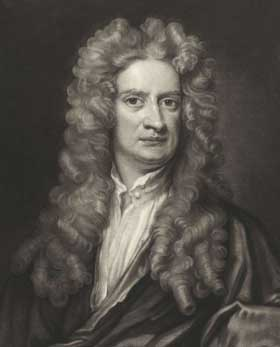# Understanding Newton's laws of motion.Sir Isaac Newton's Three Laws of Motion were first published in 1687 year and still give a fairly accurate description of nature (with a few exceptions, such as the behavior of things in distant space or inside atoms). They represent some of humanity's first great successes in applying simple mathematical formulas to describe the natural world and create an elegant and intuitive physical theory, which paved the way for later advances in physics. These laws apply to objects in the real world and have allowed us to do such things, like simulating car collisions, spaceship navigation and plays pool very well. Whether, are we aware of them, or not, Newton's laws of motion work in almost every physical activity of our daily life.

Newton's First Rule

Newton's first law says, that until the body (such as a rubber ball, car or planet) there is no force at work, the body in motion tends to stay in motion, and the body at rest tends to remain at rest. This postulate is known as the law of inertia. In practice, it means, that a rolling ball or other object slows down only because of forces such as gravity and friction. Even more intuitive, the ball at rest is not going anywhere, unless she gets poked or tossed. Considering this law, a ball thrown in the vacuum of space would theoretically travel at the same speed for that long, how long could it avoid collisions with celestial bodies and their gravity!

Newton's second law

Newton's second law is a quantitative description of change, what force it can trigger in the movement of the body. It states, that when an external force acts on the body, it accelerates (speed change) body in the direction of the force. This postulate is most often written as F = ma , where F (force) i a (acceleration) are vector quantities, therefore they are both in size, what direction, a m (time) is permanent. Although it may seem a bit confusing, Newton's second law is one of the most important in all physics and, like the first, it is also quite intuitive. For example, think about a small rubber ball and a bowling ball. To make them roll together at the same speed, you'd have to push harder (apply more force) for a bigger one, a heavier bowling ball, because it has a greater mass. Similarly, if two balls are rolling down the hill together, can be predicted, that the bowling ball will strike the wall with more destructive force than the smaller ball. It happens so, because its force is equal to the product of its mass and its acceleration.

Newton's third law

Newton's third law says, that when two bodies interact, they exert forces on themselves, which are equal in size and opposite in direction. This is commonly referred to as the law of action and reaction (colloquially speaking “each action has an equal and opposite reaction”). This idea is clearly evident in the gun's recoil: the explosion of the projectile leaving the barrel causes, that the gun moves quickly in the opposite direction. Slightly less intuitive, but the fact is also true, that the book resting on the table exerts a downward force on him, equal to its weight, and the table exerts an equal and opposite force on the book. This force is present, because the weight of the book causes a slight deformation of the table, which presses against the book like a coiled spring. If the table hadn't been able to do that, the weight of the book would break it.

Posted on Categories Education